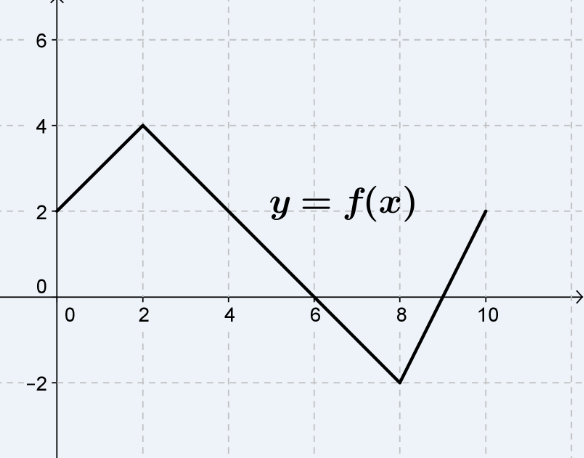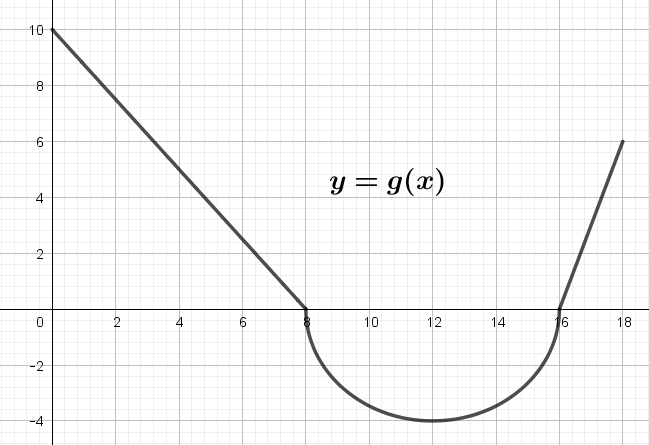### (a) $$\displaystyle{\int_{0}^4 f(x) dx}$$            (b) $$\displaystyle{\int_{6}^{10} f(x) dx}$$           (c) $$\displaystyle{\int_{0}^{10} f(x) dx}$$### 2. The graph of $$g$$ consists of two straight lines and a semicircle. Use it to evaluate each integral.  solution